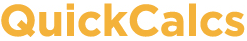1. Select category 2. Choose calculator 3. Enter data 4. View results

# Confidence interval of a proportion or count

### Confidence interval of a proportion

This is useful when you have a categorical variable with two possible outcomes, for example male vs female, pass vs fail, infected vs not infected. Enter the total number of subjects, objects or events as the denominator. For the numerator, enter the number of subjects, objects or events who had the first of the two outcomes. This calculator will compute the proportion that had the first outcome (numerator/denominator) and the 95% confidence interval of that proportion.

Numerator:    Denominator:

### Confidence interval of a count

Enter the actual number of objects you counted in a defined volume, or the actual number of events that happened in a defined period of time. This calculator will compute the 95% confidence interval for the average number of objects in that volume or the average number of events in that time period (using the Poisson distribution). Warning: Do not normalize to convenient units. Enter the number of objects (i.e. cells) or events (i.e. radioactive disintegrations) you actually counted, not the number you would have counted in some different volume or interval.

Number counted: